Choose Language Hide Translation Bar
How to stack data for a Oneway analysis

The data you want to import into JMP often requires some manipulation before it’s ready to be analyzed in JMP. Sometimes data is arranged so that a row contains information for multiple observations. To prepare your data for analysis, you must restructure it so that each row of the JMP data table contains information for a single observation. In this example, you will see how to restructure data by stacking and recoding a data table in JMP. After the data is stacked, we can perform a Oneway analysis.

The data used in this example is called Fill Weights.xlsx, which is located in the Samples/Import Data folder installed with JMP. This data represents the weights of cereal boxes produced on three production lines. The goal is to stack the data in order to compare the results of the production lines and see whether they are producing approximately the same mean fill weight. Ideally, the mean fill weight of each production line will be close to the target fill weight.

In Fill Weights.xlsx, the production lines are arranged in three sets of columns. In your JMP data table, you need to stack the data from the three production lines into a single set of columns. This way, each row represents the data for a single part.

The figure below shows the initial format of the data in Excel. The weights of cereal boxes are randomly sampled from three different production lines.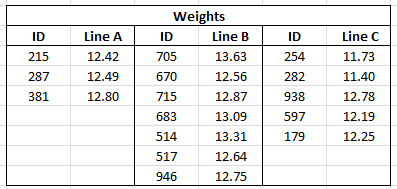The ID columns contain an identifier for each cereal box that was measured. The Line columns contain the weights (in ounces) for boxes sampled from the corresponding production line.

The target fill weight for the boxes is 12.5 ounces. Although you are interested in whether the three production lines meet the target, initially you want to see whether the three lines achieve the same mean fill rate. After the data is set up properly, you can conduct a Oneway analysis to test for differences among the mean fill weights.

Import the Data

To get started, first you must import the data. Select File > Open in JMP and select Fill Weights.xlsx from the Samples/Import Data folder.

In the Excel Import Wizard preview, row 1 contains information about the table, and row 2 is blank. The column header information starts on row 3. Also, rows 3 and 4 both contain column header information. Change the settings in the Excel Import Wizard so that the column headers start on row 3 and the number of rows with column headers is 2.

Here’s what the data table looks like once you’re finished editing in the Excel Import Wizard: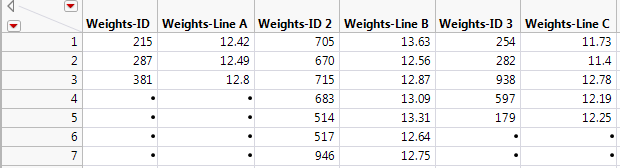Imported Data Table

The data is placed in seven rows, and multiple IDs appear in each row. For each of the three lines, there is an ID and Weight column, giving a total of six columns.

Notice that the “Weights” part of the ID column name is unnecessary and misleading. You could rename the columns now, but it will be more efficient to rename the columns after you stack the data.

Stack the Data

Reshape the data so that each row in the JMP data table reflects only a single observation. This requires you to stack the cereal box IDs, the line identifiers and the weights into columns.

To do this, select Tables > Stack to place one observation in each row of a new data table. Because you are stacking two series, ID and Line, this is a multiple series stack. In the Stack window, select the Eliminate Missing Rows option to get rid of any rows with missing data. This is the completed Stack window: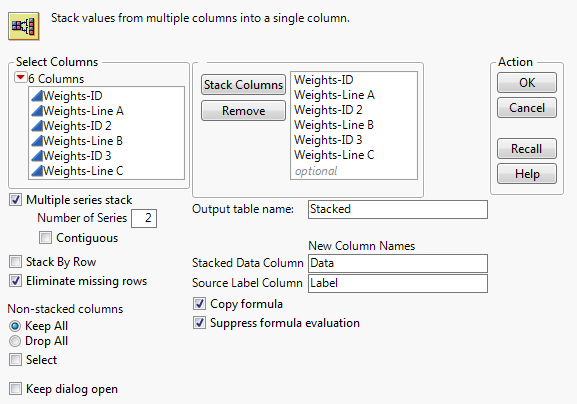Stack Window

The stacked data table contains columns labeled Data and Data 2. These columns contain the ID and Weight data. Delete the Label column since the entries were the column headings for the box IDs, which you don’t need in your table.

To make the data table more understandable, rename each column by double-clicking on the column header. In this example, the columns are renamed as follows: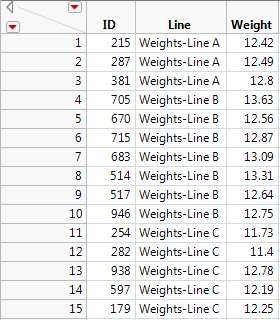As mentioned previously, you can exclude the “Weights” part of the Line column to make the table more readable. Click the Line column header to select the column and select Cols > Recode.

Change the values in the New Values column to match those in the figure below.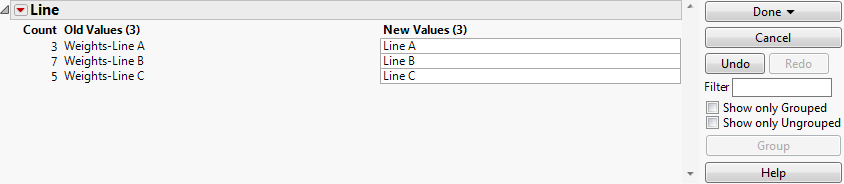Recode columns

After recoding and selecting Done > In Place, your new data table is now properly structured to analyze in JMP. Now, each row contains data for a single cereal box. The first column gives the box ID, the second gives the production line, and the third gives the weight of the box.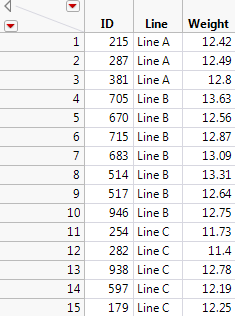Completed stacked data table

Conduct the Oneway Analysis

Now that your data is stacked, we can conduct a Oneway Analysis of Variance to test for differences in the mean fill weights among the three production lines.

To do this, select Analyze > Fit Y by X and assign Weight to Y, Response and Line to X, Factor. Once the plot is created, select Means/Anova from the red triangle menu.

The mean diamonds in the plot show 95% confidence intervals for the production line means. The points that fall outside the mean diamonds are not outliers. To see this, add box plots to the plot. From the red triangle menu, select Display Options > Box Plots.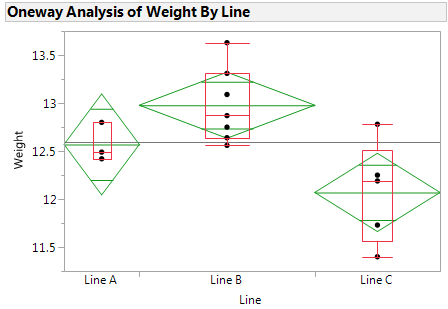Box plots

Notice all points fall within the box plots boundaries; therefore, they aren’t outliers.

Let’s look at the All Pairs, Tukey HSD comparison results. From the red triangle menu, select Compare Means > All Pairs, Tukey HSD. In the plot, click on the comparison circle for Line C. Here are the results: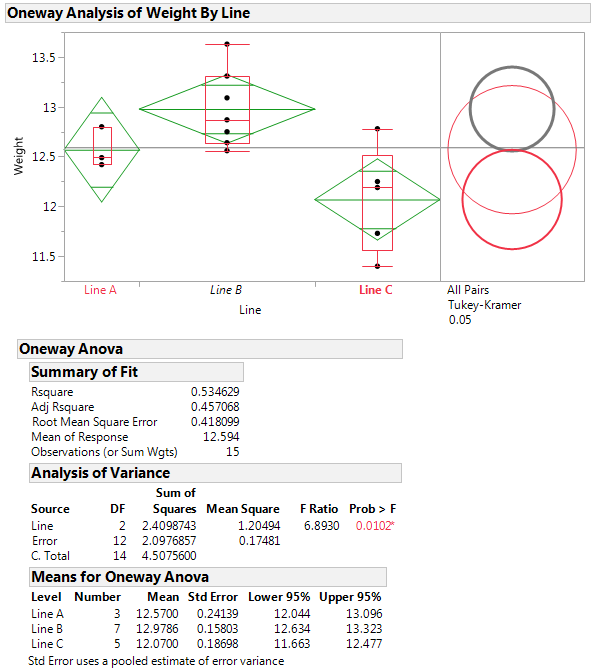Weight by line

In the Analysis of Variance report, the p-value of 0.0102 provides evidence that the means are not all equal. Compare each group means visually by examining the intersection of the comparison circles. The outside angle of intersection tells you whether the group means are significantly different. If the intersection angle is close to 90 degrees, you can verify whether the means are significantly different by clicking on the comparison circle to select it.

Groups that are different from the selected group appear as thick gray circles. Notice Line C is selected and appears red (in JMP default colors), and Line B appears as thick gray. This means Line B is not in the same group as Line A, therefore their means are significantly different. The mean for Line C differs from the mean for Line B at the 0.05 significance level. Lines A and B do not show a statistically significant difference.

In addition, the mean diamonds shown in the plot span 95% confidence intervals for the means. The numeric bounds for the 95% confidence intervals are given in the Means for Oneway ANOVA report. The plot indicates that the confidence intervals for Lines B and C do not contain the target fill weight of 12.5: Line B appears to overfill and Line C appears to underfill. For these two production lines, the underlying causes that result in off-target fill weights should be addressed. Perhaps equipment needs replacing, or maybe the lines need adjusting.

With data structured similarly to the data used in this example, whatever your case might be, stacking it for a Oneway analysis is a great way to compare your results.

Article Labels

There are no labels assigned to this post.